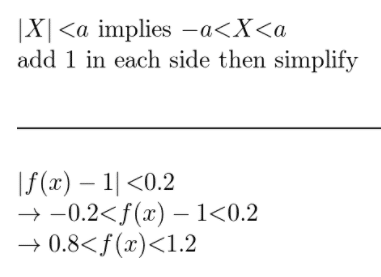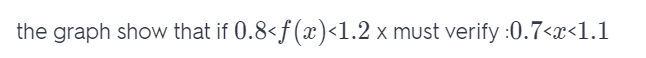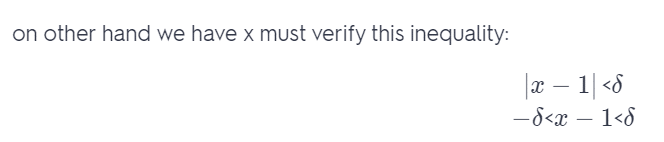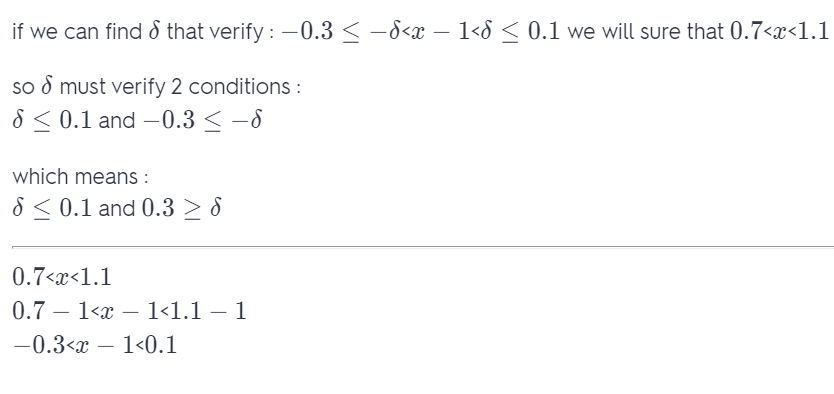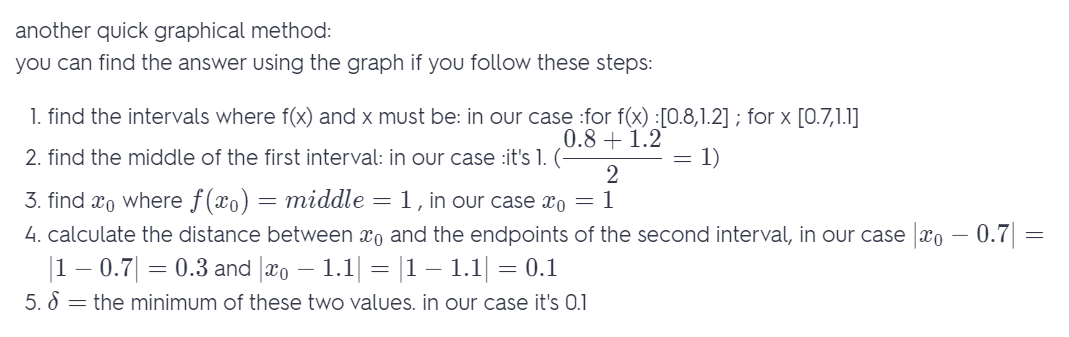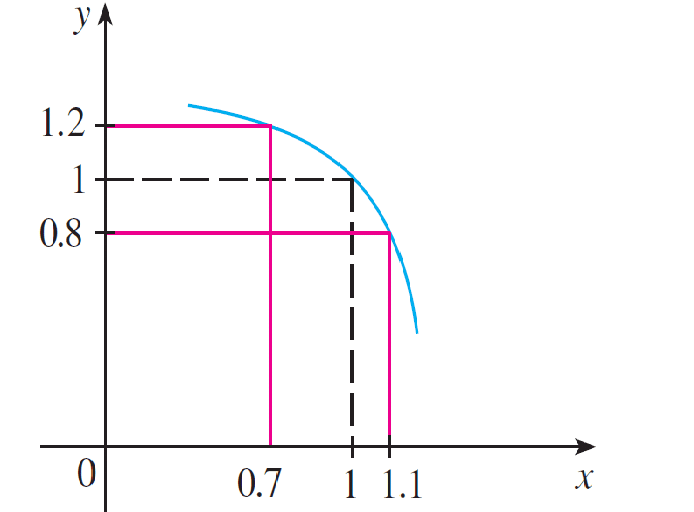# Use the Given Graph Of f To Find a Number δ Such That If |x − 1| < δ then |f(x) − 1| < 0.2

We thoroughly check each answer to a question to provide you with the most correct answers. Found a mistake? Let us know about it through the REPORT button at the bottom of the page.

Use the given graph off to find a number δ such that
if I x – 1 I < δ then I f(x) – 1 I < 0.2

## Explanation